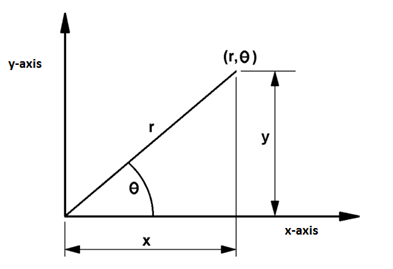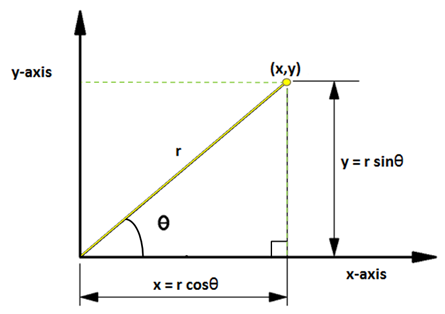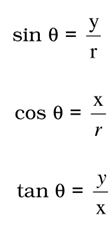# Polar Co-ordinates

The polar co-ordinate is a two-dimensional system in which each point on a plane is determined by a reference point distance and a reference direction angle. Apart from Cartesian co-ordinates, another way of locating a point on a graph uses the distance (r) from the origin and an angle (θ) measured anticlockwise from x-axis to the line joined to the origin point. These co-ordinates (r, θ) are called as polar co-ordinates.## Conversions

To convert between polar and rectangular co-ordinates, make a right triangle to the point (x,y), as shown below. The conversion formulae are derived based on this picture.Polar co-ordinates to rectangular co-ordinates

The formula to convert polar to rectangular co-ordinates.

x = r cosθ

y = r sinθ

Example

Convert the polar co-ordinates (5,30o) to rectangular co-ordinates.

(x,y)   = (5 cos30o, 5 sin30o)

= (5 x 0.87), (5 x 0.5)

= (4.3301, 2.5)

Rectangular co-ordinates to polar co-ordinates

The formula to convert rectangular to polar co-ordinates.Example

Convert the rectangular co-ordinates (4,2) to polar co-ordinates.

r = √(42 + 22)

= √20

= 4.472

tanθ = 2/4

= 0.5

θ = 26.57o (from tangent table)